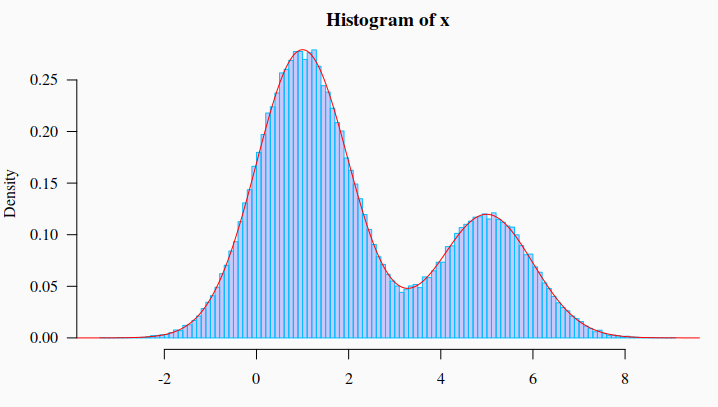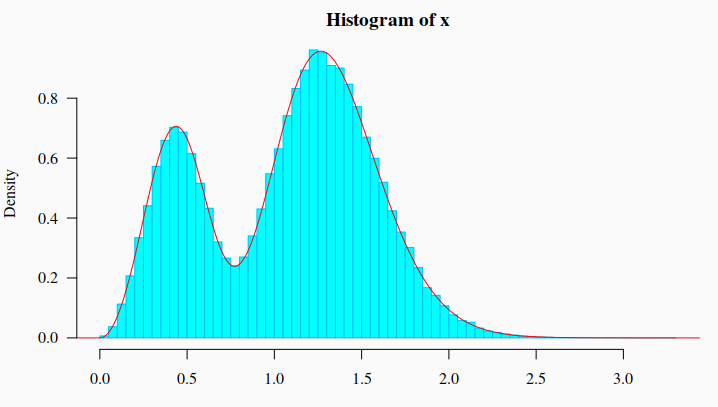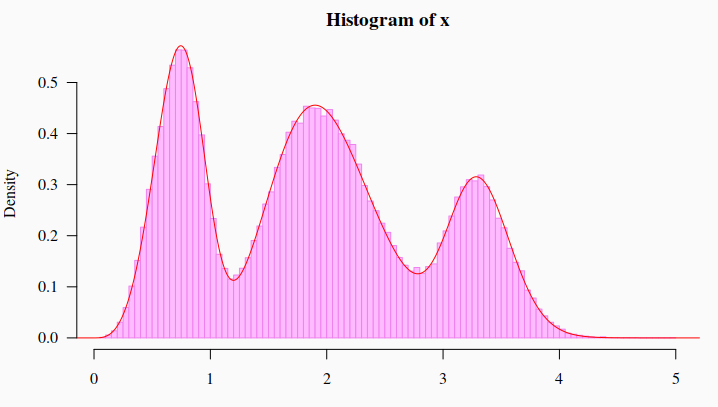## Sampling from a Mixture of Distributions

It is said that a distribution $f(x)$ is a mixture of k components distributions $f_1(x), …, f_k(x)$ if:

$f(x) = \sum_{i=1}^k \pi_i f_i(x)$

where $\pi_i$ are the so called mixing weights, $0 \le \pi_i \le 1$, and $\pi_1 + … + \pi_k = 1$. Here, new data points from distribution will be generated in the standard way: first to pick a distribution, with probabilities given by the mixing weights, and then to generate one observation according to that distribution. More information about mixture distribution can be read in Wikipedia.

# 1. Generating random variables from a mixture of normal distributions

To generate from a mixture distribution the R package usefr will be used.

library(usefr)set.seed(123) # set a seed for random generation# ========= A mixture of three distributions =========phi = c(7/10, 3/10) # Mixture proportions# ---------------------------------------------------------

# === Named vector of the corresponding distribution function parameters
# must be provided
args <- list(norm = c(mean = 1, sd = 1), norm = c(mean = 5, sd = 1))# ------------------------------------------------------------
# ===== Sampling from the specified mixture distribution ====
x <- rmixtdistr(n = 1e5, pi = pi , arg = args)# ------------------------------------------------------------
# === The graphics for the simulated dataset and the corresponding theoretical
# mixture distribution
par(bg = "gray98", mar = c(3, 4, 2, 1) )
hist(x, 90, freq = FALSE, las = 1, family = "serif", col = rgb(0, 0, 1, 0.2), border = "deepskyblue")
x1 <- seq(-4, 10, by = 0.001)
lines(x1, dmixtdistr(x1, phi = phi, arg = args), col = "red")# 2. Mixture of Weibull and Gamma distributions

Mixture of normal distributions is what most frequently we see online and in paper. Let’s see the mixture of Weibull and Gamma distributions.

set.seed(123) # set a seed for random generation # ==== A mixture of three distributions ===== pi = c(7/10, 3/10) # Mixture proportions
# ---------------------------------------------------------
# === Named vector of the corresponding distribution function parameters # must be provided args <- list(gamma = c(shape = 20, scale = 1/15), weibull = c(shape = 3, scale = 0.5)) # --------------------------------------------------------- # === Sampling from the specified mixture distribution ==== x <- rmixtdistr(n = 1e5, pi = pi , arg = args) # --------------------------------------------------------- # === The graphics for the simulated dataset and the corresponding theoretical # mixture distribution par(bg = "gray98", mar = c(3, 4, 2, 1) ) hist(x, 90, freq = FALSE, las = 1, family = "serif", col = "cyan1", border = "deepskyblue") x1 <- seq(-4, 10, by = 0.001) lines(x1, dmixtdistr(x1, pi = pi, arg = args), col = "red")# 3. Mixture of Gamma, Weibull, and Log-Normal distributions

set.seed(123) # set a seed for random generation# =============== A mixture of three distributions ========================pi = c(5/10, 3/10, 2/10) # Mixture proportions# --------------------------------------------------------------------------# ==== Named vector of the corresponding distribution function parameters# must be providedargs <- list(gamma = c(shape = 20, scale = 1/10),             weibull = c(shape =  4, scale = 0.8),             lnorm = c(meanlog = 1.2, sdlog = 0.08))# --------------------------------------------------------------------------# ======= Sampling from the specified mixture distribution =======x <- rmixtdistr(n = 1e5, pi = pi , arg = args)# --------------------------------------------------------------------------# The graphics for the simulated dataset and the corresponding theoretical# mixture distributionpar(bg = "gray98",  mar = c(3, 4, 2, 1) )hist(x, 90, freq = FALSE, las = 1, family = "serif", col = "plum1", border = "violet")x1 <- seq(-4, 10, by = 0.001)lines(x1, dmixtdistr(x1, pi = pi, arg = args), col = "red")